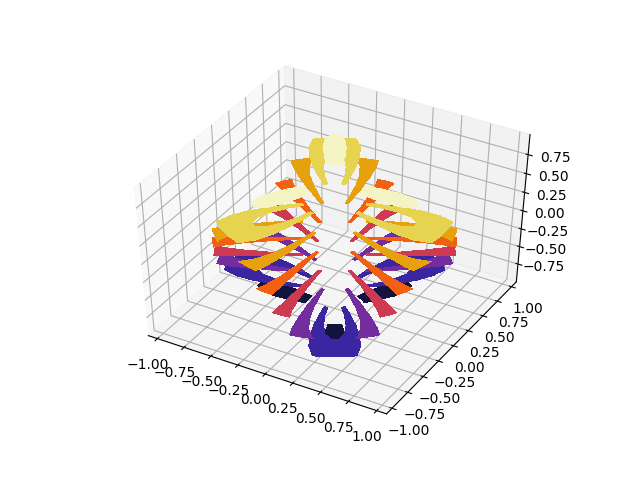# Triangular 3D filled contour plot¶

Filled contour plots of unstructured triangular grids.

The data used is the same as in the second plot of trisurf3d_demo2. tricontour3d_demo shows the unfilled version of this example.# This import registers the 3D projection, but is otherwise unused.
from mpl_toolkits.mplot3d import Axes3D  # noqa: F401 unused import

import matplotlib.pyplot as plt
import matplotlib.tri as tri
import numpy as np

# First create the x, y, z coordinates of the points.
n_angles = 48

# Create the mesh in polar coordinates and compute x, y, z.
angles = np.linspace(0, 2*np.pi, n_angles, endpoint=False)
angles = np.repeat(angles[..., np.newaxis], n_radii, axis=1)
angles[:, 1::2] += np.pi/n_angles

# Create a custom triangulation.
triang = tri.Triangulation(x, y)

y[triang.triangles].mean(axis=1))